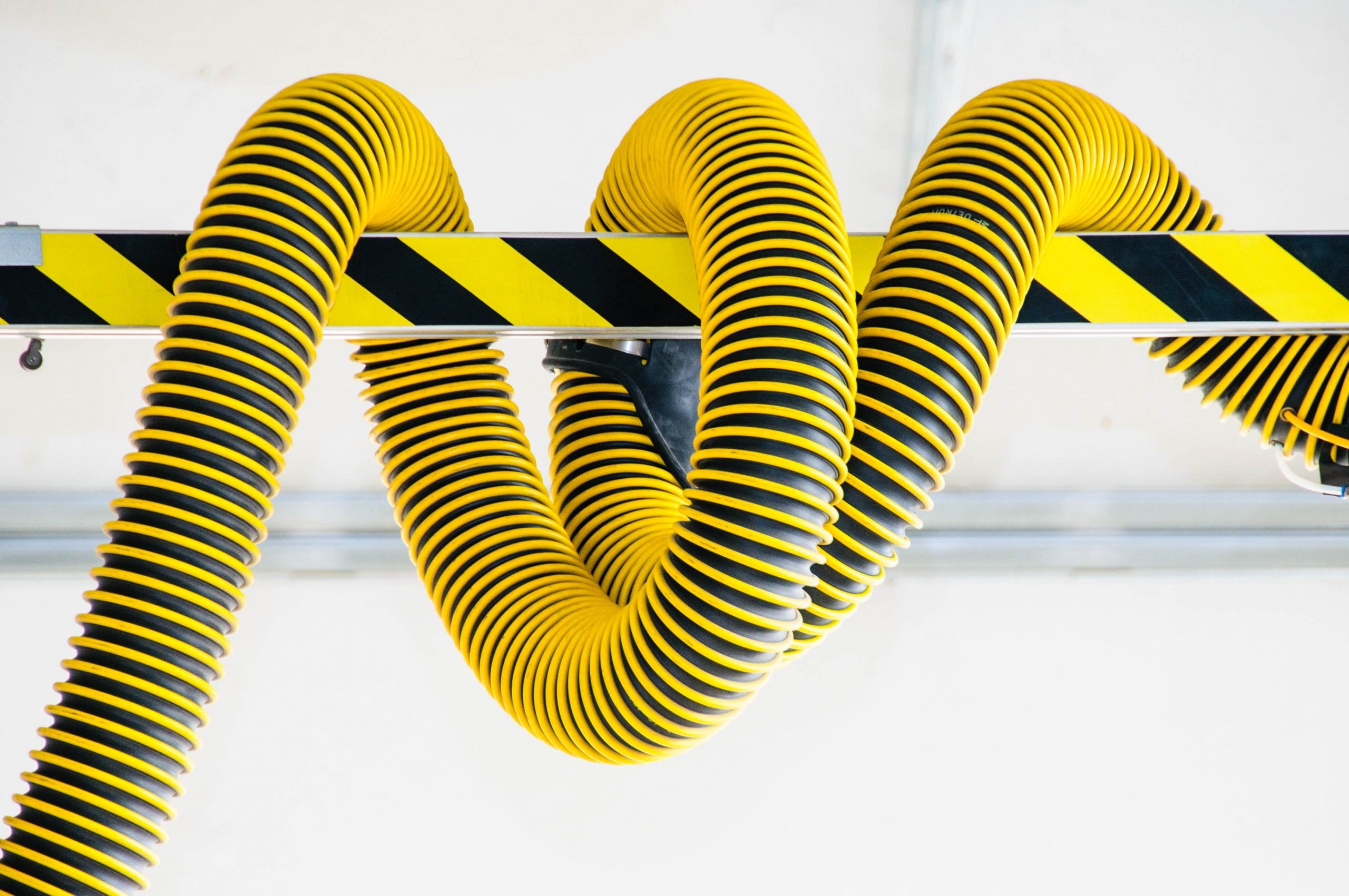# How to Delete, Clear, Erase A C++ Vector Member On WindowsWhat are the Vectors in C++? How can I delete a vector member in C++? How can we use pop_back() method in vectors? How can we use clear() method in vectors? How can we use erase() method in vectors? Let’s answer these questions.

Arrays and structs in C++ are explained well in our previous posts. These were coming from the C, in this post, we will explain another great feature of C++, The Vectors.

## What is a C++ vector?

Vectors are dynamic arrays included in <vector> library in modern C++ and they can resize themselves automatically when a member of a vector is inserted or deleted. Vectors are the same as dynamic arrays and these dynamic arrays of vectors are handled automatically by the container. Vectors are the way of Modern C++, their members are placed in the contiguous memory storage, thus it can be resized, and it can be accessed and traversed using iterators.

When we Insert data into vectors, this may take a time than other static arrays because of the need of extending the vector array. Vectors have low memory usage as in dynamic array implementations, because of having good data cache utilization and locality of reference. We can easily access an element of a vector by giving its index between ‘[‘ and ‘]’ as same as arrays, which means vector members can be referenced by indices.

Vectors allow random access; that is, an element of a vector may be referenced in the same manner as elements of arrays (by array indices). Linked lists and sets, on the other hand, do not support random access or pointer arithmetic. Vectors are very useful for storing data in lists whose number of elements (length in total) may not be known before setting up the list. Because the vector data structure allocates the necessary memory needed for specific data storage Erasing and clearing vector elements from a vector does not need to free any of the memory associated with that element. That makes vectors much more safe and modern in C++ than arrays.

## How do I define a vector in C++?

A vector can be defined with this syntax,

Now let’s see how we can add and delete a vector member.

### How can I delete the last C++ vector member?

We can add a new member of vector by using push_back() and we can delete the last member of vector by using pop_back() method of vector. Here is an example,

### How do I erase a C++ vector member with an Iterator Index?

We can erase vector member by using erase() method of vector. We can use Iterator index, to point the address of that vector we should add vec.begin() to the index. Here is the example below,

### How can I delete all C++ vector members?

We can delete all vector members by using resize() method of vector. Here is an example,

### How can I delete a C++ vector definition?

We can delete vector definition from the memory by using clear() method of vector. Thus we can use more memory after we finish about the all process of the vector data. Here is how we can use it.

If you click on the following dynamic search link you will see other articles on LearnCPlusPlus in connection with vectors: https://learncplusplus.org/?s=vectors

C++ Builder is the easiest and fastest C and C++ IDE for building simple or professional applications on the Windows, MacOS, iOS & Android operating systems. It is also easy for beginners to learn with its wide range of samples, tutorials, help files, and LSP support for code. RAD Studio’s C++ Builder version comes with the award-winning VCL framework for high-performance native Windows apps and the powerful FireMonkey (FMX) framework for cross-platform UIs.

There is a free C++ Builder Community Edition for students, beginners, and startups; it can be downloaded from here. For professional developers, there are Professional, Architect, or Enterprise versions of C++ Builder and there is a trial version you can download from here.